## Example Questions

### Example Question #1 : How To Find A Ratio Of Exponents

If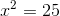and, then which of the following CANNOT be the value of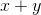?Explanation:

Even roots of numbers can either be positive or negative. Thus, x = +/- 5 and y = +/- 3. The possible values from x + y can therefore be:

(-5) + (-3) = -8

(-5) + 3 = -2

5 + (-3) = 2

5 + 3 = 8

### Example Question #1 : Exponential Ratios

If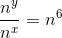for allnot equal to 0, which of the following must be true?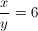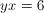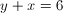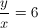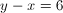Explanation:

Remember that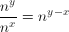Since the problem states that, you can assume that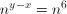This shows that.

### Example Question #1 : How To Find A Ratio Of Exponents

Ifandare positive integers and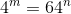, then what is the value of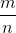?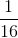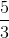Explanation:

43 = 64

Alternatively written, this is 4(4)(4) = 64 or 43 = 641.

Thus, m = 3 and n = 1.

m/n = 3/1 = 3.

### Example Question #1 : How To Find A Ratio Of Exponents

Write the following logarithm in expanded form: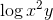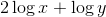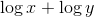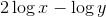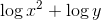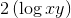Explanation: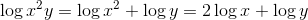### Example Question #3 : How To Find A Ratio Of Exponents

If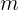andare both rational numbers and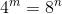, what is?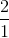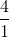Explanation:

This question is asking you for the ratio of m to n.  To figure it out, the easiest way is to figure out when 4 to an exponent equals 8 to an exponent.  The easiest way to do that is to list the first few results of 4 to an exponent and 8 to an exponent and check to see if any match up, before resorting to more drastic means of finding a formula.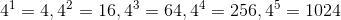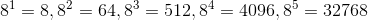And, would you look at that.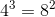.  Therefore,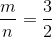.